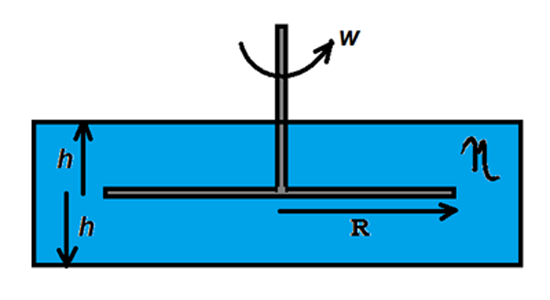# Liquid Friction!A Thin circular disk of radius $R$, rotates in a cylindrical chamber, which is filled with water (coefficient of viscosity $\eta$). It is rotated with constant angular velocity $\omega$.
Find the Power developed (in watts)by the viscous forces, if the distance between the top-bottom walls and the disk is $h$.

Details and Assumptions:
$\bullet$ Neglect end effects.
$\bullet$ $\eta = 8.9\times 10^{-4}$
$\bullet$ $R = 2m$
$\bullet$ $h = 1mm$
$\bullet$ $w = 2 rad/s$

×